# 大神十年编程经验，200篇详细教程，教你从零到一学Python（万字总结之机器学习篇）

[Python从零到壹] 十二.机器学习之回归分析万字总结（线性回归、多项式回归、逻辑回归） | 【生长吧！Python】

# 一.回归

## 1.什么是回归• 给出一个点集，构造一个函数来拟合这个点集，并且尽可能的让该点集与拟合函数间的误差最小，如果这个函数曲线是一条直线，那就被称为线性回归，如果曲线是一条三次曲线，就被称为三次多项回归。

## 2.线性回归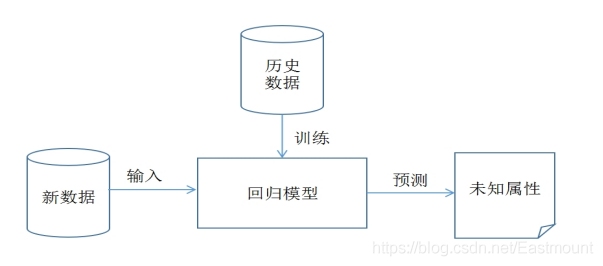# 二.线性回归分析

## 1.LinearRegression

LinearRegression回归模型在Sklearn.linear_model子类下，主要是调用fit(x,y)函数来训练模型，其中x为数据的属性，y为所属类型。sklearn中引用回归模型的代码如下：

``````from sklearn import linear_model          #导入线性模型
regr = linear_model.LinearRegression()    #使用线性回归
print(regr)
``````

``````LinearRegression(copy_X=True,
fit_intercept=True,
n_jobs=1,
normalize=False)
``````

LinearRegression类主要包括如下方法：• fit(X,y[,n_jobs])
对训练集X，y进行训练，分析模型参数，填充数据集。其中X为特征，y为标记或类属性。
• predict(X)
使用训练得到的估计器或模型对输入的X数据集进行预测，返回结果为预测值。数据集X通常划分为训练集和测试集。
• decision_function(X)
使用训练得到的估计器或模型对数据集X进行预测。它与predict(X)区别在于该方法包含了对输入数据的类型检查和当前对象是否存在coef_属性的检查，更安全。
• score(X, y[,]samples_weight)
返回对于以X为samples、y为target的预测效果评分。
• get_params([deep])
获取该估计器（Estimator）的参数。
• **set_params(params)
设置该估计器（Estimator）的参数。
• coef_
存放LinearRegression模型的回归系数。
• intercept_
存放LinearRegression模型的回归截距。

``````# -*- coding: utf-8 -*-
# By:Eastmount CSDN 2021-07-03
from sklearn import linear_model     #导入线性模型
import matplotlib.pyplot as plt
import numpy as np

#X表示企业成本 Y表示企业利润
X = [, , , , , , , , , , , , , , ]
Y = [, , , , , , , , , , , , , , ]
print('数据集X: ', X)
print('数据集Y: ', Y)

#回归训练
clf = linear_model.LinearRegression()
clf.fit(X, Y)

#预测结果
X2 = [, , ]
Y2 = clf.predict(X2)
print(Y2)
res = clf.predict(np.array().reshape(-1, 1))
print('预测成本1200元的利润：\$%.1f' % res)

#绘制线性回归图形
plt.plot(X, Y, 'ks')                 #绘制训练数据集散点图
plt.plot(X2, Y2, 'g-')               #绘制预测数据集直线
plt.show()
``````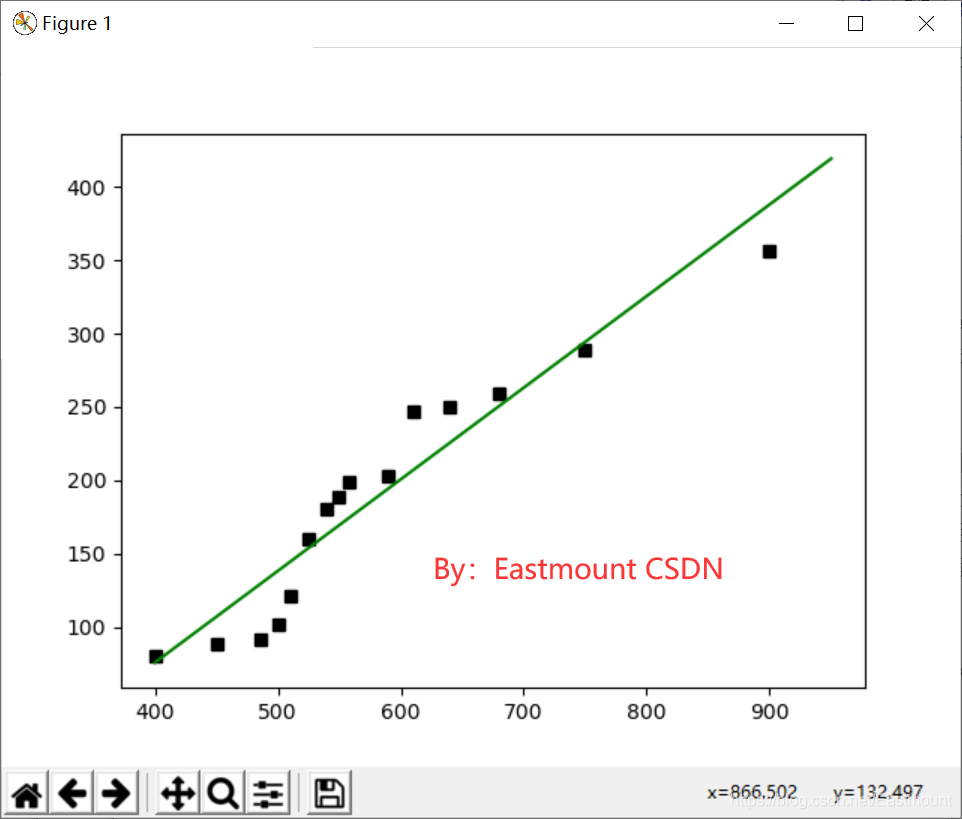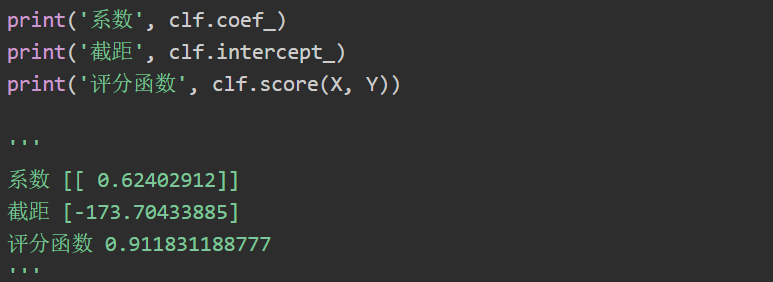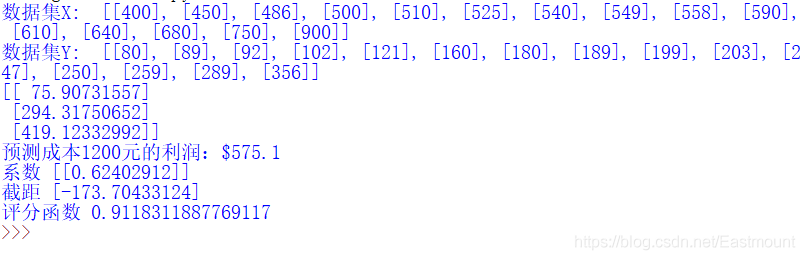## 2.线性回归预测糖尿病

(1).糖尿病数据集
Sklearn机器学习包提供了糖尿病数据集（Diabetes Dataset），该数据集主要包括442行数据，10个特征值，分别是：年龄（Age）、性别（Sex）、体质指数（Body mass index）、平均血压（Average Blood Pressure）、S1~S6一年后疾病级数指标。预测指标为Target，它表示一年后患疾病的定量指标。原网址的描述如图4所示：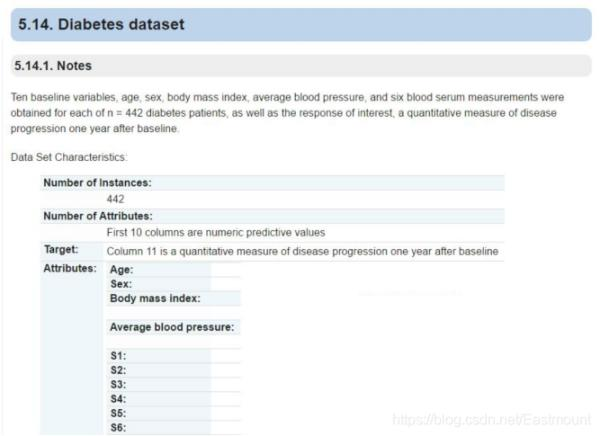``````# -*- coding: utf-8 -*-
# By:Eastmount CSDN 2021-07-03
from sklearn import datasets
print(diabetes.data)                                          #数据
print(diabetes.target)                                        #类标
print('总行数: ', len(diabetes.data), len(diabetes.target))
print('特征数: ', len(diabetes.data))                      #每行数据集维数
print('数据类型: ', diabetes.data.shape)
print(type(diabetes.data), type(diabetes.target))
``````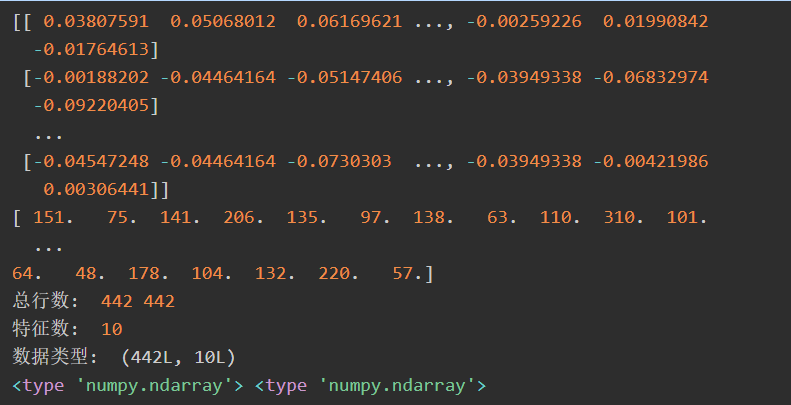(2).代码实现

``````# -*- coding: utf-8 -*-
# By:Eastmount CSDN 2021-07-03
from sklearn import datasets
import matplotlib.pyplot as plt
from sklearn import linear_model
import numpy as np

#数据集划分
diabetes_x_temp = diabetes.data[:, np.newaxis, 2]  #获取其中一个特征
diabetes_x_train = diabetes_x_temp[:-20]           #训练样本
diabetes_x_test = diabetes_x_temp[-20:]            #测试样本 后20行
diabetes_y_train = diabetes.target[:-20]           #训练标记
diabetes_y_test = diabetes.target[-20:]            #预测对比标记

#回归训练及预测
clf = linear_model.LinearRegression()
clf.fit(diabetes_x_train, diabetes_y_train)        #训练数据集
pre = clf.predict(diabetes_x_test)

#绘图
plt.title(u'LinearRegression Diabetes')            #标题
plt.xlabel(u'Attributes')                          #x轴坐标
plt.ylabel(u'Measure of disease')                  #y轴坐标
plt.scatter(diabetes_x_test, diabetes_y_test, color = 'black')  #散点图
plt.plot(diabetes_x_test, pre, color='blue', linewidth = 2)     #预测直线
plt.show()
``````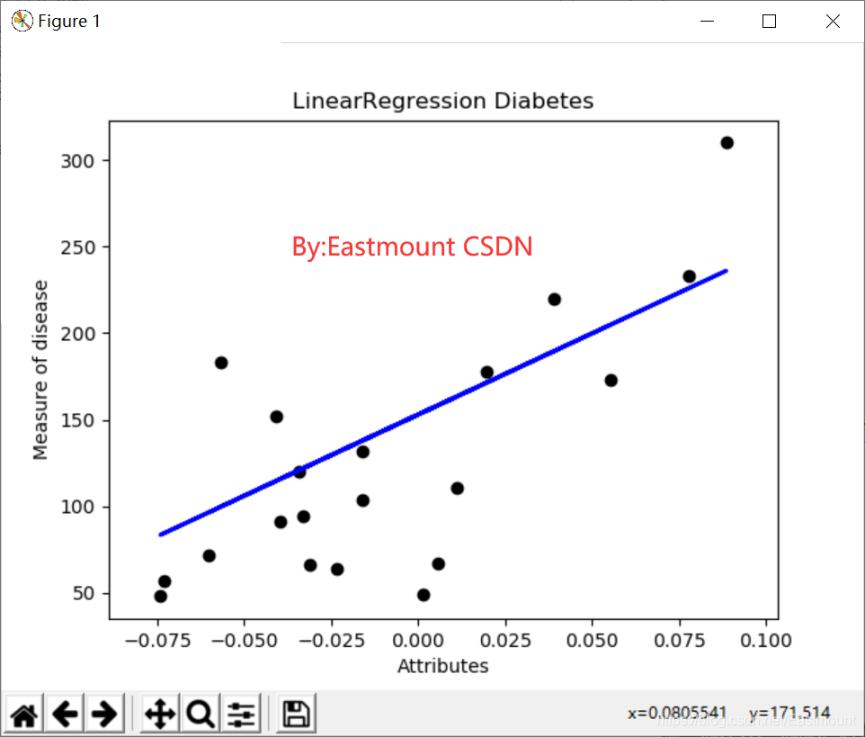(3).代码优化

``````# -*- coding: utf-8 -*-
# By:Eastmount CSDN 2021-07-03
from sklearn import datasets
import numpy as np
from sklearn import linear_model
import matplotlib.pyplot as plt

#第一步 数据集划分
x = d.data
x_one = x[:,np.newaxis, 2]    #获取一个特征 第3列数据
y = d.target                  #获取的正确结果
x_train = x_one[:-42]         #训练集X [  0:400]
x_test = x_one[-42:]          #预测集X [401:442]
y_train = y[:-42]             #训练集Y [  0:400]
y_test = y[-42:]              #预测集Y [401:442]

#第二步 线性回归实现
clf = linear_model.LinearRegression()
print(clf)
clf.fit(x_train, y_train)
pre = clf.predict(x_test)
print('预测结果', pre)
print('真实结果', y_test)

#第三步 评价结果
cost = np.mean(y_test-pre)**2   #2次方
print('平方和计算:', cost)
print('系数', clf.coef_)
print('截距', clf.intercept_)
print('方差', clf.score(x_test, y_test))

#第四步 绘图
plt.plot(x_test, y_test, 'k.')      #散点图
plt.plot(x_test, pre, 'g-')        #预测回归直线
#绘制点到直线距离
for idx, m in enumerate(x_test):
plt.plot([m, m],[y_test[idx], pre[idx]], 'r-')

plt.savefig('blog12-01.png', dpi=300) #保存图片
plt.show()
``````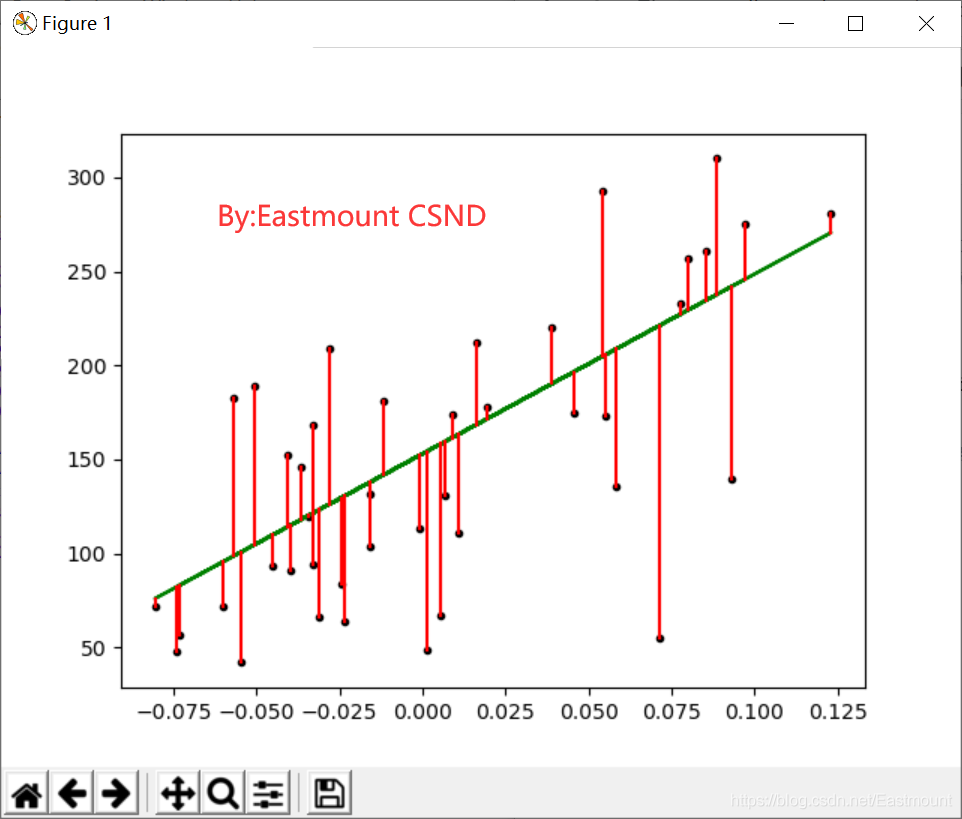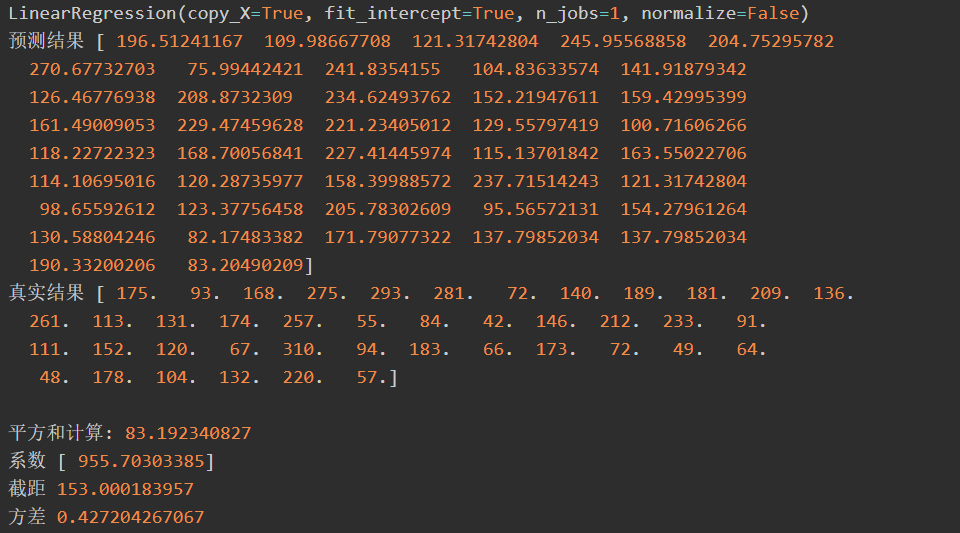# 三.多项式回归分析

## 1.基础概念## 2.PolynomialFeatures

Python的多项式回归需要导入sklearn.preprocessing子类中PolynomialFeatures类实现。PolynomialFeatures对应的函数原型如下：

``````class sklearn.preprocessing.PolynomialFeatures(degree=2,
interaction_only=False,
include_bias=True)
``````

PolynomialFeatures类在Sklearn官网给出的解释是：专门产生多项式的模型或类，并且多项式包含的是相互影响的特征集。共有三个参数，degree表示多项式阶数，一般默认值是2；interaction_only如果值是true（默认是False），则会产生相互影响的特征集；include_bias表示是否包含偏差列。

PolynomialFeatures类通过实例化一个多项式，建立等差数列矩阵，然后进行训练和预测，最后绘制相关图形，接下来与前面的一元线性回归分析进行对比试验。

## 3.多项式回归预测成本和利润

``````# -*- coding: utf-8 -*-
# By:Eastmount CSDN 2021-07-03
from sklearn.linear_model import LinearRegression
from sklearn.preprocessing import PolynomialFeatures
import matplotlib.pyplot as plt
import numpy as np

#X表示企业成本 Y表示企业利润
X = [, , , , , , , , , , , , , , ]
Y = [, , , , , , , , , , , , , , ]
print('数据集X: ', X)
print('数据集Y: ', Y)

#第一步 线性回归分析
clf = LinearRegression()
clf.fit(X, Y)
X2 = [, , ]
Y2 = clf.predict(X2)
print(Y2)
res = clf.predict(np.array().reshape(-1, 1))
print('预测成本1200元的利润：\$%.1f' % res)
plt.plot(X, Y, 'ks')    #绘制训练数据集散点图
plt.plot(X2, Y2, 'g-')  #绘制预测数据集直线

#第二步 多项式回归分析
xx = np.linspace(350,950,100) #350到950等差数列
quadratic_featurizer = PolynomialFeatures(degree = 2) #实例化一个二次多项式

#把训练好X值的多项式特征实例应用到一系列点上,形成矩阵
label="\$y = ax^2 + bx + c\$",linewidth=2)
plt.legend()
plt.show()
``````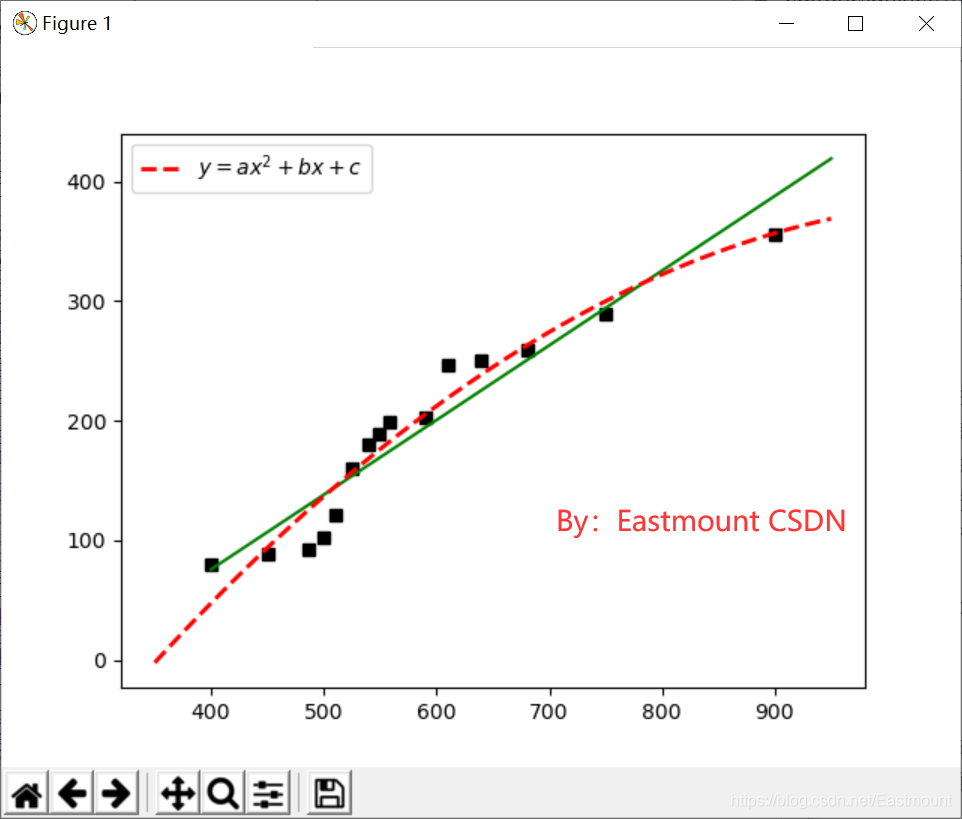``````print('1 r-squared', clf.score(X, Y))
``````

``````('1 r-squared', 0.9118311887769025)
('2 r-squared', 0.94073599498559335)
``````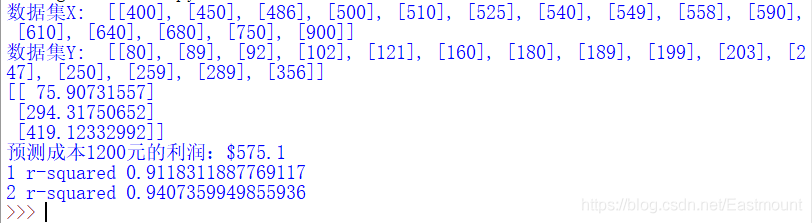``````# -*- coding: utf-8 -*-
# By:Eastmount CSDN 2021-07-03
from sklearn.linear_model import LinearRegression
from sklearn.preprocessing import PolynomialFeatures
import matplotlib.pyplot as plt
import numpy as np

#X表示企业成本 Y表示企业利润
X = [, , , , , , , , , , , , , , ]
Y = [, , , , , , , , , , , , , , ]
print('数据集X: ', X)
print('数据集Y: ', Y)

#第一步 线性回归分析
clf = LinearRegression()
clf.fit(X, Y)
X2 = [, , ]
Y2 = clf.predict(X2)
print(Y2)
res = clf.predict(np.array().reshape(-1, 1))
print('预测成本1200元的利润：\$%.1f' % res)
plt.plot(X, Y, 'ks')    #绘制训练数据集散点图
plt.plot(X2, Y2, 'g-')  #绘制预测数据集直线

#第二步 多项式回归分析
xx = np.linspace(350,950,100)
#把训练好X值的多项式特征实例应用到一系列点上,形成矩阵
label="\$y = ax^2 + bx + c\$",linewidth=2)
plt.legend()
plt.show()
print('1 r-squared', clf.score(X, Y))

# ('1 r-squared', 0.9118311887769025)
# ('5 r-squared', 0.98087802460869788)
``````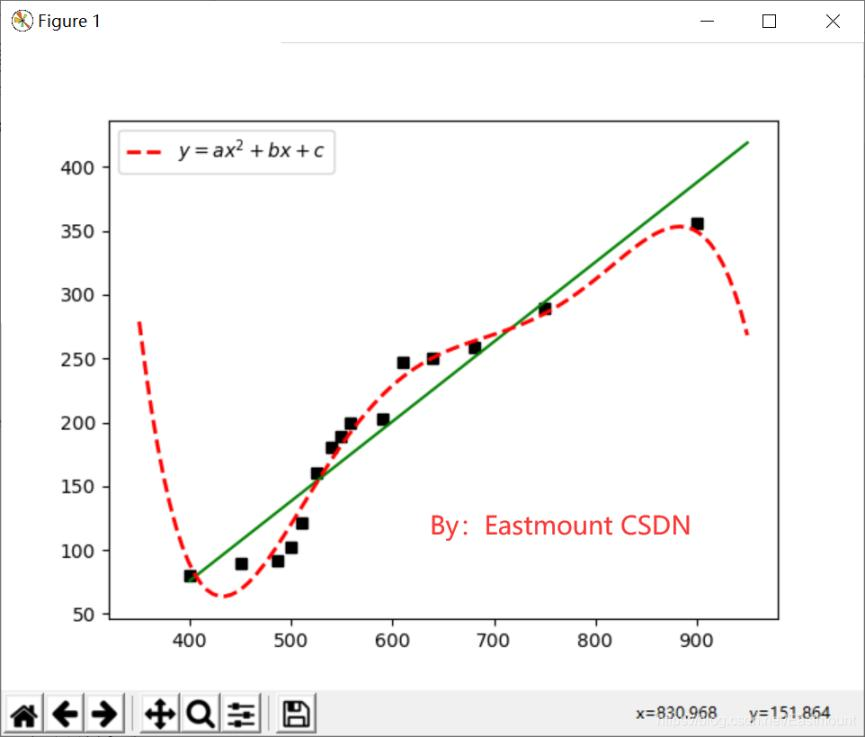# 四.逻辑回归

## 1.基础原理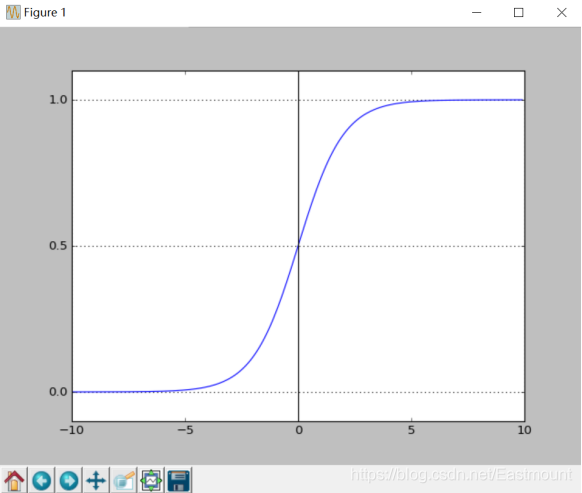y=0的概率分布公式定义如下：``````# -*- coding: utf-8 -*-
# By:Eastmount CSDN 2021-07-03
import matplotlib.pyplot as plt
import numpy as np

def Sigmoid(x):
return 1.0 / (1.0 + np.exp(-x))

x= np.arange(-10, 10, 0.1)
h = Sigmoid(x)                #Sigmoid函数
plt.plot(x, h)
plt.axvline(0.0, color='k')   #坐标轴上加一条竖直的线（0位置）
plt.axhspan(0.0, 1.0, facecolor='1.0', alpha=1.0, ls='dotted')
plt.axhline(y=0.5, ls='dotted', color='k')
plt.yticks([0.0, 0.5, 1.0])  #y轴标度
plt.ylim(-0.1, 1.1)          #y轴范围
plt.show()
``````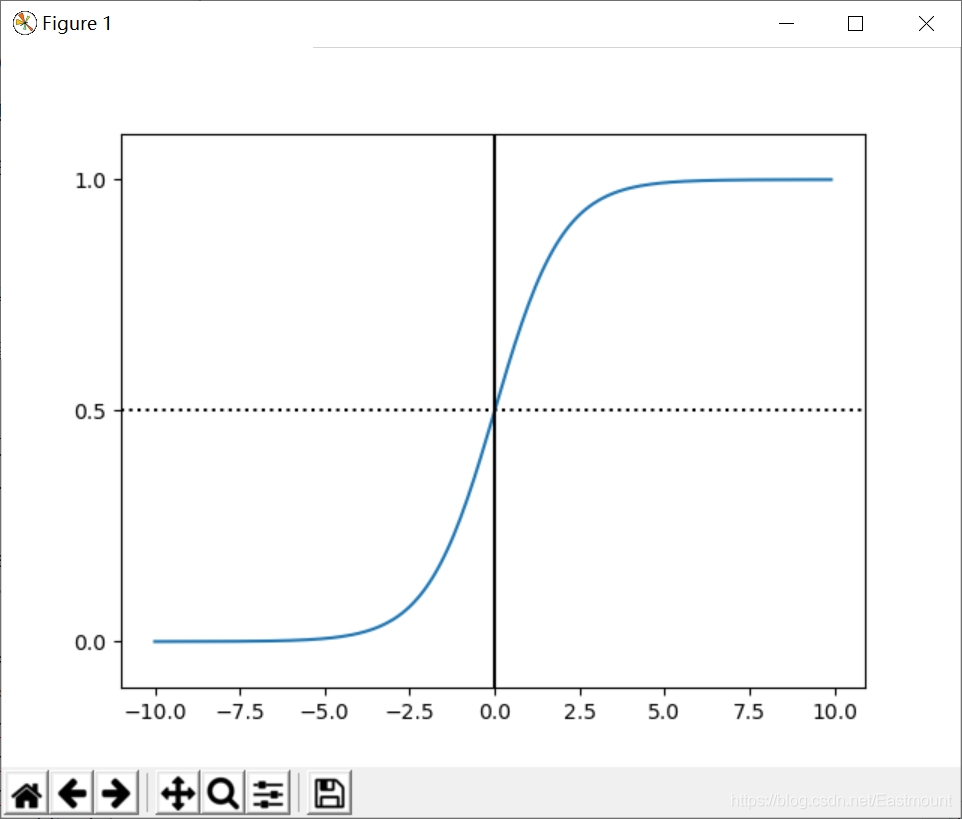## 2.LogisticRegression

LogisticRegression回归模型在Sklearn.linear_model子类下，调用sklearn逻辑回归算法步骤比较简单，即：

• 导入模型。调用逻辑回归LogisticRegression()函数。
• fit()训练。调用fit(x,y)的方法来训练模型，其中x为数据的属性，y为所属类型。
• predict()预测。利用训练得到的模型对数据集进行预测，返回预测结果。

``````# -*- coding: utf-8 -*-
# By:Eastmount CSDN 2021-07-03
from sklearn.linear_model import LogisticRegression  #导入逻辑回归模型
clf = LogisticRegression()
print(clf)
clf.fit(train_feature,label)
predict['label'] = clf.predict(predict_feature)
``````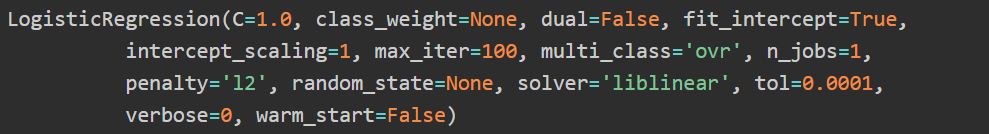## 3.鸢尾花数据集回归分析实例

(1).鸢尾花数据集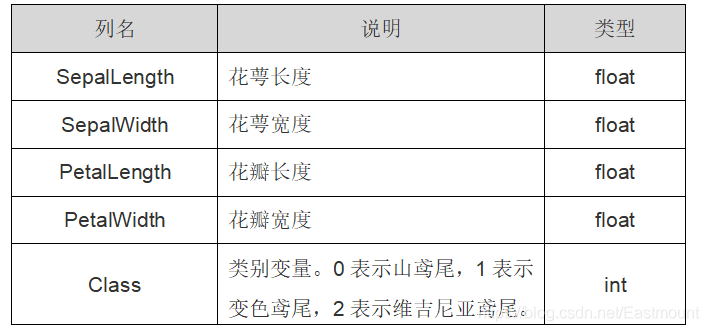Class 类别变量。0表示山鸢尾，1表示变色鸢尾，2表示维吉尼亚鸢尾。 int
iris里有两个属性iris.data，iris.target。data是一个矩阵，每一列代表了萼片或花瓣的长宽，一共4列，每一行代表一个被测量的鸢尾植物，一共采样了150条记录，即150朵鸢尾花样本。

``````from sklearn.datasets import load_iris   #导入数据集iris
print(iris.data)
``````
target是一个数组，存储了每行数据对应的样本属于哪一类鸢尾植物，要么是山鸢尾（值为0），要么是变色鸢尾（值为1），要么是维吉尼亚鸢尾（值为2），数组的长度是150。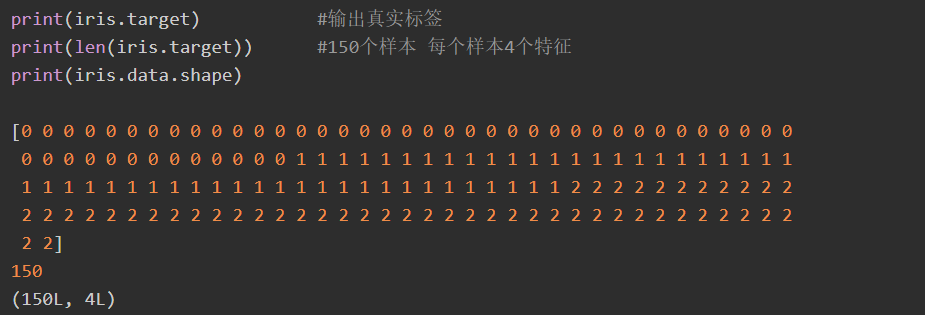(2).散点图绘制

``````# -*- coding: utf-8 -*-
# By:Eastmount CSDN 2021-07-03
import matplotlib.pyplot as plt
import numpy as np

#载入数据集
print(iris.data)           #输出数据集
print(iris.target)         #输出真实标签

#获取花卉两列数据集
DD = iris.data
X = [x for x in DD]
print(X)
Y = [x for x in DD]
print(Y)

#plt.scatter(X, Y, c=iris.target, marker='x')
plt.scatter(X[:50], Y[:50], color='red', marker='o', label='setosa') #前50个样本
plt.scatter(X[50:100], Y[50:100], color='blue', marker='x', label='versicolor') #中间50个
plt.scatter(X[100:], Y[100:],color='green', marker='+', label='Virginica') #后50个样本
plt.legend(loc=2) #左上角
plt.show()
``````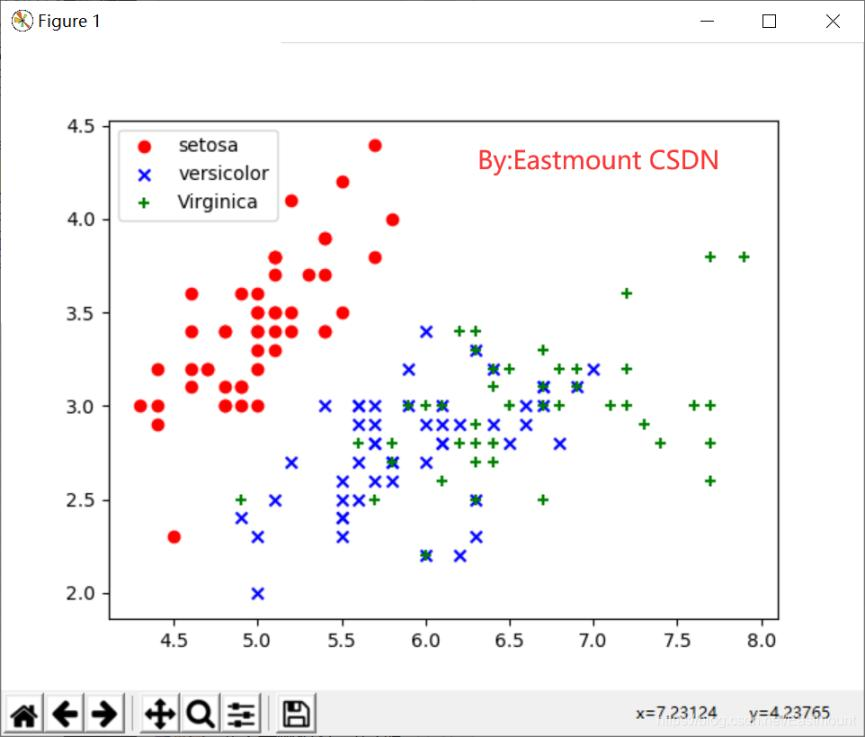(3).线性回归分析

``````# -*- coding: utf-8 -*-
# By:Eastmount CSDN 2021-07-03

#第一步 导入数据集

#获取花瓣的长和宽
x = [n for n in hua.data]
y = [n for n in hua.data]
import numpy as np #转换成数组
x = np.array(x).reshape(len(x),1)
y = np.array(y).reshape(len(y),1)

#第二步 线性回归分析
from sklearn.linear_model import LinearRegression
clf = LinearRegression()
clf.fit(x,y)
pre = clf.predict(x)
print(pre)

#第三步 画图
import matplotlib.pyplot as plt
plt.scatter(x,y,s=100)
plt.plot(x,pre,"r-",linewidth=4)
for idx, m in enumerate(x):
plt.plot([m,m],[y[idx],pre[idx]], 'g-')
plt.show()
``````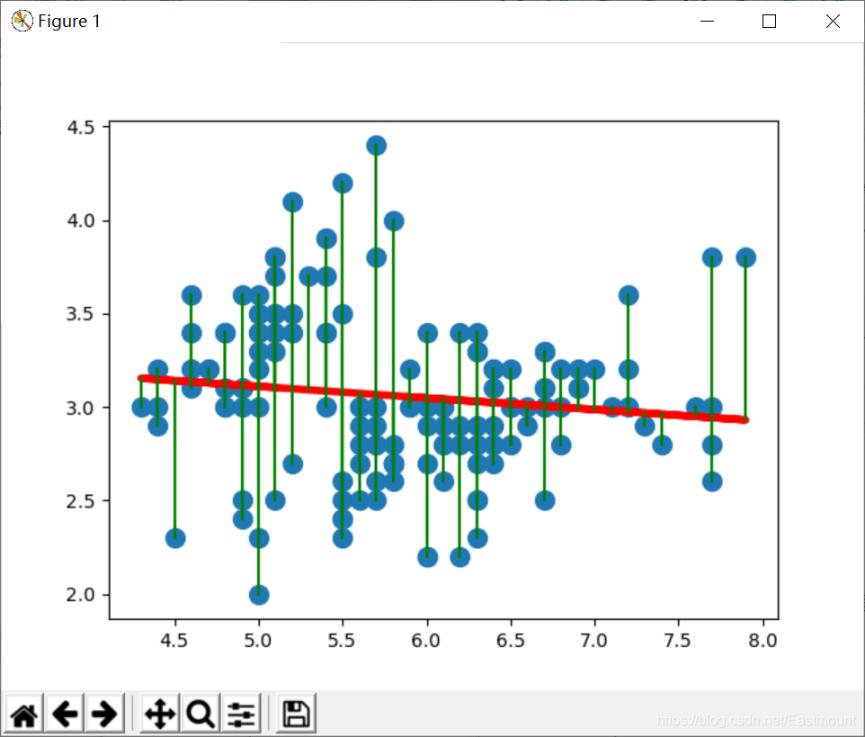(4).逻辑回归分析鸢尾花

``````# -*- coding: utf-8 -*-
# By:Eastmount CSDN 2021-07-03
import matplotlib.pyplot as plt
import numpy as np
from sklearn.linear_model import LogisticRegression

#载入数据集
X = X = iris.data[:, :2]   #获取花卉两列数据集
Y = iris.target

#逻辑回归模型
lr = LogisticRegression(C=1e5)
lr.fit(X,Y)

#meshgrid函数生成两个网格矩阵
h = .02
x_min, x_max = X[:, 0].min() - .5, X[:, 0].max() + .5
y_min, y_max = X[:, 1].min() - .5, X[:, 1].max() + .5
xx, yy = np.meshgrid(np.arange(x_min, x_max, h), np.arange(y_min, y_max, h))

#pcolormesh函数将xx,yy两个网格矩阵和对应的预测结果Z绘制在图片上
Z = lr.predict(np.c_[xx.ravel(), yy.ravel()])
Z = Z.reshape(xx.shape)
plt.figure(1, figsize=(8,6))
plt.pcolormesh(xx, yy, Z, cmap=plt.cm.Paired)

#绘制散点图
plt.scatter(X[:50,0], X[:50,1], color='red',marker='o', label='setosa')
plt.scatter(X[50:100,0], X[50:100,1], color='blue', marker='x', label='versicolor')
plt.scatter(X[100:,0], X[100:,1], color='green', marker='s', label='Virginica')

plt.xlabel('Sepal length')
plt.ylabel('Sepal width')
plt.xlim(xx.min(), xx.max())
plt.ylim(yy.min(), yy.max())
plt.xticks(())
plt.yticks(())
plt.legend(loc=2)
plt.show()
``````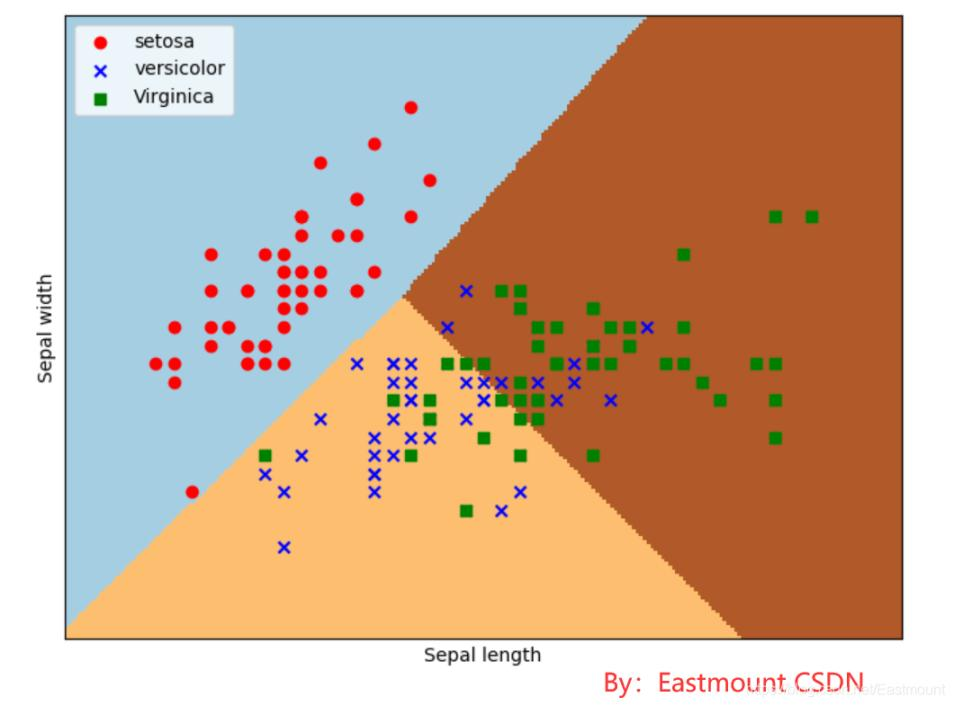• lr = LogisticRegression(C=1e5)
初始化逻辑回归模型，C=1e5表示目标函数。
• lr.fit(X,Y)
调用逻辑回归模型进行训练，参数X为数据特征，参数Y为数据类标。
• xx, yy = np.meshgrid(np.arange(x_min, x_max, h), np.arange(y_min, y_max, h))
获取鸢尾花数据集的两列数据，对应为花萼长度和花萼宽度，每个点的坐标就是(x,y)。 先取X二维数组的第一列（长度）的最小值、最大值和步长h（设置为0.02）生成数组，再取X二维数组的第二列（宽度）的最小值、最大值和步长h生成数组， 最后用meshgrid函数生成两个网格矩阵xx和yy，如下所示：
• Z = lr.predict(np.c_[xx.ravel(), yy.ravel()])
调用ravel()函数将xx和yy的两个矩阵转变成一维数组，由于两个矩阵大小相等，因此两个一维数组大小也相等。np.c_[xx.ravel(), yy.ravel()]是获取并合并成矩阵，即：

• Z = logreg.predict(np.c_[xx.ravel(), yy.ravel()])
调用predict()函数进行预测，预测结果赋值给Z。即：
• Z = Z.reshape(xx.shape)
调用reshape()函数修改形状，将Z变量转换为两个特征（长度和宽度），则39501个数据转换为171*231的矩阵。Z = Z.reshape(xx.shape)输出如下：
• plt.pcolormesh(xx, yy, Z, cmap=plt.cm.Paired)
调用pcolormesh()函数将xx、yy两个网格矩阵和对应的预测结果Z绘制在图片上，可以发现输出为三个颜色区块，分布表示分类的三类区域。cmap=plt.cm.Paired表示绘图样式选择Paired主题，输出区域如下图所示：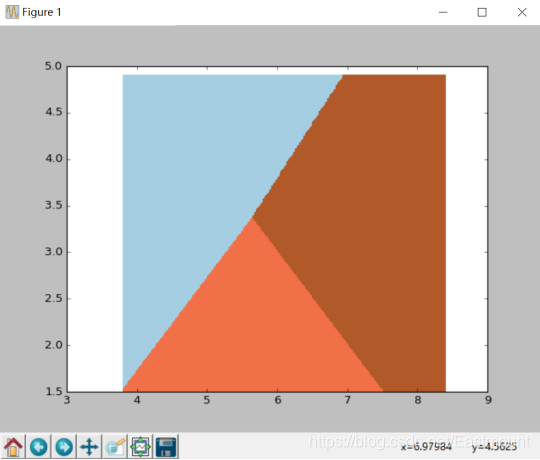• plt.scatter(X[:50,0], X[:50,1], color=‘red’,marker=‘o’, label=‘setosa’)
调用scatter()绘制散点图，第一个参数为第一列数据（长度），第二个参数为第二列数据（宽度），第三、四个参数为设置点的颜色为红色，款式为圆圈，最后标记为setosa。

0个评论
• 消灭零评论